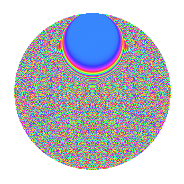# Properties

 Label 1849.2.kLevel $1849$ Weight $2$ Character orbit 1849.k Rep. character $\chi_{1849}(6,\cdot)$ Character field $\Q(\zeta_{129})$ Dimension $13188$ Sturm bound $315$

# Related objects

## Defining parameters

 Level: $$N$$ $$=$$ $$1849 = 43^{2}$$ Weight: $$k$$ $$=$$ $$2$$ Character orbit: $$[\chi]$$ $$=$$ 1849.k (of order $$129$$ and degree $$84$$) Character conductor: $$\operatorname{cond}(\chi)$$ $$=$$ $$1849$$ Character field: $$\Q(\zeta_{129})$$ Sturm bound: $$315$$

## Dimensions

The following table gives the dimensions of various subspaces of $$M_{2}(1849, [\chi])$$.

Total New Old
Modular forms 13356 13356 0
Cusp forms 13188 13188 0
Eisenstein series 168 168 0

## Trace form

 $$13188q - 82q^{2} - 88q^{3} - 398q^{4} - 89q^{5} - 83q^{6} - 87q^{7} - 68q^{8} + 67q^{9} + O(q^{10})$$ $$13188q - 82q^{2} - 88q^{3} - 398q^{4} - 89q^{5} - 83q^{6} - 87q^{7} - 68q^{8} + 67q^{9} - 79q^{10} - 78q^{11} - 84q^{12} - 85q^{13} - 168q^{14} - 89q^{15} - 418q^{16} - 81q^{17} - 82q^{18} + 132q^{19} - 103q^{20} - 114q^{21} - 96q^{22} + 5q^{23} - 90q^{24} + 66q^{25} - 101q^{26} - 76q^{27} - 80q^{28} - 77q^{29} + 212q^{30} + 6q^{31} - 66q^{32} - 76q^{33} - 72q^{34} - 412q^{35} + 49q^{36} - 98q^{37} - 7q^{38} - 66q^{39} - 57q^{40} - 88q^{41} - 84q^{42} - 52q^{43} - 94q^{44} - 118q^{45} - 112q^{46} - 96q^{47} - 99q^{48} + 74q^{49} - 103q^{50} - 64q^{51} - 62q^{52} - 9q^{53} - 94q^{54} - 86q^{55} - 89q^{56} + 46q^{57} - 92q^{58} - 116q^{59} - 59q^{60} - 100q^{61} - 81q^{62} - 109q^{63} - 416q^{64} - 76q^{65} - 808q^{66} - 89q^{67} + 438q^{68} - 107q^{69} - 88q^{70} - 84q^{71} - 1257q^{72} - 78q^{73} - 84q^{74} - 780q^{75} + 103q^{76} - 71q^{77} - 172q^{78} - 92q^{79} - 113q^{80} + 71q^{81} + 460q^{82} - 73q^{83} - 66q^{84} - 128q^{85} - 117q^{86} - 1298q^{87} - 96q^{88} - 84q^{89} - 1606q^{90} - 106q^{91} - 51q^{92} - 81q^{93} + 488q^{94} + 126q^{95} - 52q^{96} - 451q^{97} - 70q^{98} + 124q^{99} + O(q^{100})$$

## Decomposition of $$S_{2}^{\mathrm{new}}(1849, [\chi])$$ into newform subspaces

The newforms in this space have not yet been added to the LMFDB.

## Hecke characteristic polynomials

There are no characteristic polynomials of Hecke operators in the database